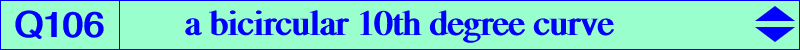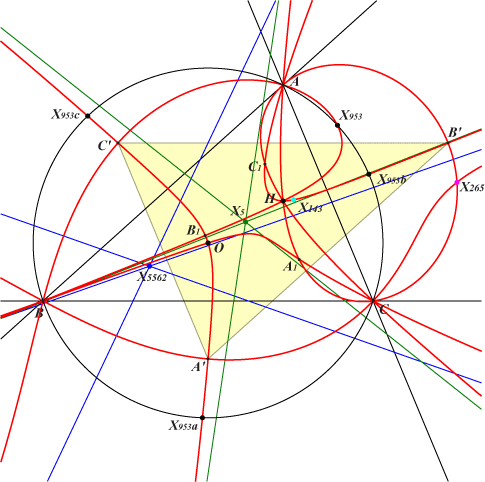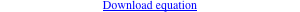too complicated to be written here. Click on the link to download a text file.X(3), X(4), X(143), X(265), X(953) extraversions of X(953) infinite points of the McCay cubic infinite points of the cevian lines of X(3) other points belowQ106 is related to Q023, see locus property 3. Q106 is a bicircular curve of degree 10. It has 6 real asymptotes namely the parallels at X(5562) to the cevian lines of O and the parallels at X(5) to the asymptotes of the McCay cubic K003. Q106 meets the circumcircle (O) at 20 points namely the vertices of ABC (each counting for 4), the circular points at infinity (each counting for 2), X(953) and its extraversions labelled X953a, X953b, X953c. The orthocenter H = X(4) is a quadruple point on the curve. Q106 has 8 other double points namely : the circumcenter O = X(3) with nodal tangents parallel to the asymptotes of the Jerabek hyperbola, X(265) the reflections A', B', C' of O in the sidelines of ABC or equivalently the reflections of A, B, C about X(5), A1, B1, C1 on the cevian lines of X(143). Following Q023, locus property 3, one (or several) point P on Q023 corresponds to a point Q on Q106. Here are some examples :P on Q023 Q on Q106 remarks in/excenters X(4) X(3) X(3) X(3) is a node on both curves with same nodal tangents X(36) X(953) extraversions of X(36) extraversions of X(953) X(54) X(143) X(2574), X(2575) X(265) A, B, C infinite points of the cevian lines of O vertices of the circumnormal triangle infinite points of the asymptotes of K003 2 points on BC apart B, C node A' nodes B', C' likewise 2 points on the parallel at O to BC 2 points on AH, symmetric about BC 4 other points on BH, CH likewise 2 points on AX(54) apart A, X(54) node A1 on AX(143) nodes B1, C1 likewise